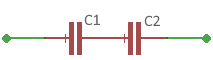# Capacitor series

Calculator and formula for calculating the capacity of a series circuit

## Calculate capacitor series circuit

This function calculates the total capacitance or a series capacitor in a series circuit of two capacitors.

For the calculation, select whether the total capacitance or the capacitance of capacitor C2 should be calculated. Then enter the values and click on the Calculate button.

Capacity calculator

 What should be calculated? Total capacity Capacitor C2 Input Capacitor C1 pF nF µF mF F Total capacity pF nF µF mF F Capacitor C2 pF nF µF mF F Decimal places 0 1 2 3 4 6 8 10 Result Total capacity Capacity C2For series circuits with more than 2 capacitors, use the function here

## Capacitors in series formulas

The capacitance of two capacitors in series is calculated using the following formula

$$\displaystyle C_{ges}=\frac{C_1· C_2}{C_1 + C_2}$$

To calculate a series capacitor for a given total capacitance and given C1, use the following formula

$$\displaystyle C_2=\frac{C_1· C_{ges}}{C_1 - C_{ges}}$$